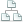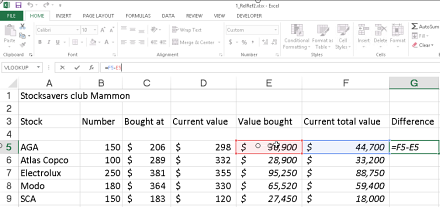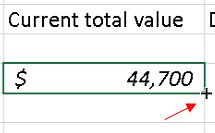Home >Tips >Excel formulas for stocksSite map

Excel Formulas for Calculating Stocks Outcome

An Excel tutorial by Peter KalmstromThe possibility to tell Excel to calculate is a crucial feature and the most important reason to use it. Peter Kalmstrom has explains how to use Excel formulas in various demos in this Tips section.

In the demo below he takes a different angle and shows some simple formulas that are especially useful when you want to calculate the result of your affairs on the stock market.When you buy stocks you want their value to raise, so the difference between the purchase price and the current value is the most important.

This is how Peter calculates the profit or loss:
1. Calculate the purchase value by multiplying the purchase price per stock with the number of stocks bought.
2. Calculate the current value by multiplying the current price per stock with the number of stocks bought.
3. Calculate the difference between the purchase value and the current value.
When this is done for one type of stocks, you can easily copy the formulas to the other stock types. Peter shows how you easily can drag relative formulas down and make them valid for more cells.Peter uses Excel 2013 for his demo, but  the formulas he uses works for earlier versions of Excel too.